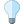You are viewing the RapidMiner Studio documentation for version 9.2 - Check here for latest version

#Decision Tree (Weight-Based) (RapidMiner Studio Core)

## Synopsis

This operator generates a pruned decision tree based on an arbitrary attribute relevance test. The attribute weighting scheme should be provided as inner operator. This operator can be applied only on ExampleSets with nominal data.

## Description

The Decision Tree (Weight-Based) operator is a nested operator i.e. it has a subprocess. The subprocess must have an attribute weighting scheme i.e. an operator that expects an ExampleSet and generates attribute weights. You need to have basic understanding of subprocesses in order to apply this operator. Please study the documentation of the Subprocess operator for basic understanding of subprocesses.

The Decision Tree (Weight-Based) operator works exactly like the Decision Tree operator with one exception: it uses an arbitrary attribute relevance test criterion instead of the information gain or gain ratio criteria. Moreover this operator cannot be applied on ExampleSets with numerical attributes. It is recommended that you study the documentation of the Decision Tree operator for basic understanding of decision trees.

If the Weight by Chi Squared Statistic operator is supplied for attribute weighting, this operator acts as the CHAID operator. CHAID stands for CHi-squared Automatic Interaction Detection. The chi-square statistic is a nonparametric statistical technique used to determine if a distribution of observed frequencies differs from the theoretical expected frequencies. Chi-square statistics use nominal data, thus instead of using means and variances, this test uses frequencies. CHAID's advantages are that its output is highly visual and easy to interpret. Because it uses multiway splits by default, it needs rather large sample sizes to work effectively, since with small sample sizes the respondent groups can quickly become too small for reliable analysis.

The representation of the data as Tree has the advantage compared with other approaches of being meaningful and easy to interpret. The goal is to create a classification model that predicts the value of the label based on several input attributes of the ExampleSet. Each interior node of the tree corresponds to one of the input attributes. The number of edges of an interior node is equal to the number of possible values of the corresponding input attribute. Each leaf node represents a value of the label given the values of the input attributes represented by the path from the root to the leaf. This description can be easily understood by studying the Example Process of the Decision Tree operator.

Pruning is a technique in which leaf nodes that do not add to the discriminative power of the decision tree are removed. This is done to convert an over-specific or over-fitted tree to a more general form in order to enhance its predictive power on unseen datasets. Pre-pruning is a type of pruning performed parallel to the tree creation process. Post-pruning, on the other hand, is done after the tree creation process is complete.

## Differentiation

###CHAID

If the Weight by Chi Squared Statistic operator is applied for attribute weighting in the subprocess of the Decision Tree (Weight-Based) operator, it works exactly like the CHAID operator.

## Input

•training set (IOObject)

This input port expects an ExampleSet. It is the output of the Generate Nominal Data operator in the attached Example Process. The output of other operators can also be used as input. This operator cannot handle numerical data, therefore the ExampleSet should not have numerical attributes.

## Output

•model (Decision Tree)

The Decision Tree is delivered from this output port. This classification model can now be applied on unseen data sets for the prediction of the label attribute.

## Parameters

• minimal_size_for_splitThe size of a node in a Tree is the number of examples in its subset. The size of the root node is equal to the total number of examples in the ExampleSet. Only those nodes are split whose size is greater than or equal to the minimal size for split parameter. Range: integer
• minimal_leaf_sizeThe size of a leaf node in a Tree is the number of examples in its subset. The tree is generated in such a way that every leaf node subset has at least the minimal leaf size number of instances. Range: integer
• maximal_depthThe depth of a tree varies depending upon size and nature of the ExampleSet. This parameter is used to restrict the size of the Decision Tree. The tree generation process is not continued when the tree depth is equal to the maximal depth. If its value is set to '-1', the maximal depth parameter puts no bound on the depth of the tree, a tree of maximum depth is generated. If its value is set to '1', a Tree with a single node is generated. Range: integer
• confidenceThis parameter specifies the confidence level used for the pessimistic error calculation of pruning. Range: real
• no_pruningBy default the Decision Tree is generated with pruning. Setting this parameter to true disables the pruning and delivers an unpruned Tree. Range: boolean
• number_of_prepruning_alternativesAs prepruning runs parallel to the tree generation process, it may prevent splitting at certain nodes when splitting at that node does not add to the discriminative power of the entire tree. In such a case alternative nodes are tried for splitting. This parameter adjusts the number of alternative nodes tried for splitting when the split is prevented by prepruning at a certain node. Range: integer

## Tutorial Processes

### Introduction to the Decision Tree (Weight-Based) operator

The Generate Nominal Data operator is used for generating an ExampleSet with 100 examples. There are three nominal attributes in the ExampleSet and every attribute has three possible values. A breakpoint is inserted here so that you can have a look at the ExampleSet. The Decision Tree (Weight-Based) operator is applied on this ExampleSet with default values of all parameters. The resultant model is connected to the result port of the process and it can be seen in the Results Workspace.